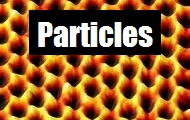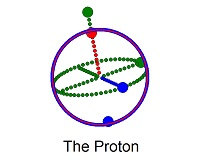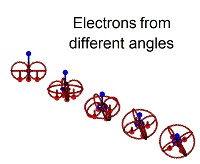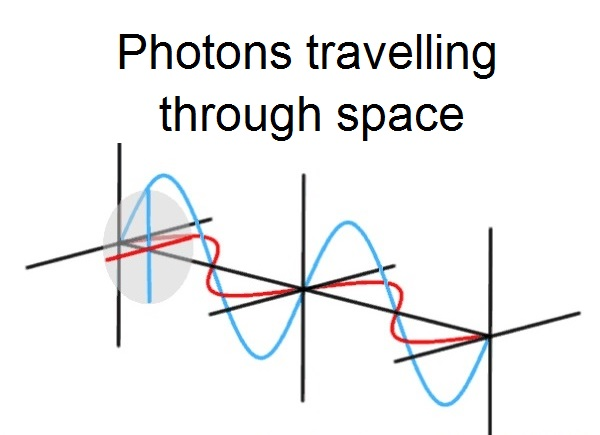Video Samples

 The Bohr radius According to 2006 CODATA the Bohr radius of hydrogen has a value of 5.2917720859(36) × 10^-11 m (i.e., approximately 53 pm or 0.53 ångströms or 53000 fm). This value can be computed in terms of other physical constants including the permittivity of free space, the speed of light in vacuum and other constants found in nature.     The force holding a single electron to a single proton never grows higher then the value calculated with coulombs law at this distance.

 The classical electron radius The classical electron radius has a value of 2.8179402894(58) x 10^-15 (i.e., approximately 3 fm or 0.003 pm). Also known as the Lorentz radius or the Thomson scattering length it is based on a classical relativistic model of the electron. Its value is calculated as based on the electric charge, the mass of the electron, the speed of light, and e0 the permittivity of free space.

 The speed of light Equals 299,792,458 meters/sec (300 picometers/attosecond). One lightyear equals 9.4605284*10^15 meters.

 The Planck constant Equals 6.626*10^-34 Joule-Sec. and establishes the relationship between length and energy. A short string = high energy (ultraviolet) and a long string = low energy (heat).      To convert from length to energy, take 1240/(nanometer length) to get (evolt energy), Ie. For red light (656nm wavelength) 1240/656nm=1.89ev

Computer Animations

Computer modeling and animation software requires that a position of electrons and protons be fixed in space and obey rules that determine where things will be over time.

This model derives a world made up of tiny electrons and large empty proton shells. Using the Shell theorem as support, a world is modelled where the electrons do not feel an attraction to the proton once inside the proton shell.

The electron is not expected to spiral into the proton while emitting radiation since it feels no force from the proton once inside the shell. Since neither the proton or the electron are point charges, we do not see any effects of an uncertainty principle and are able to track particle positions, velocities and forces with as much accuracy as is desired. The Pauli exclusion principle is a natural conseqence of this model as the charge force limits the number of electrons in the various energy orbitals.

 The Particles of Animated Physics

# The principle particles of Animated Physics

### 1.   The Neutron - charge zero, large mass 2.   The Proton - charge of plus one, large mass 3.   Electrons - charge of minus one, small mass 4.   and Photons - no charge, no mass### 1.   The Neutron - charge zero, large mass (940 MeV)

• Three quarks acting as empty spheres one inside each other, using gluons as poles for each spin axis;
• Gluons come in eight different styles and act as poles to hold quarks together and for the quarks to spin around;
• Two down quarks (-1/3 e) and one up quark (2/3 e) for net zero charge;
• The coordinated spins of three spin 1/2 quarks, results in a spin 1/2 particle with a magnetic moment of -1.9μN.

Modelled as a 3-layered spinning bloch sphere with a radius of 53 picometers and a thickness just under 1.0 femtometers. The layers spin at the speed of light with a green gravitational axis. Each red and blue layer represents an up or down quark. The neutron exists as a free particle configured as down/down/up which decays in time to a proton and an electron. It also exists in a very stable form as a down/up/down particle that acts as an insulater between layers of protons for building of elements. More..### 2.   The Proton - charge of plus one, large mass (938 MeV)

• Three quarks acting as empty spheres one inside each other, using gluons as poles for each spin axis;
• Gluons come in eight different styles and act as poles to hold quarks together and for the quarks to spin around;
• One down quark (-1/3 e) and two up quarks (2/3 e) for net plus one charge;
• The coordinated spins of three spin 1/2 quarks, results in a spin 1/2 particle with a magnetic moment of 2.8μN.

Like the neutron, the proton is modelled as a 3-layered spinning bloch sphere with a radius of 53 picometers. The proton is created through the decay of a neutron ending up with an up/down/up quark configuration with a positive charge. Elements are created as layers of proton shells, insulated with neutron shells.

The proton's charge is located on the shell with measureable differences in energy levels inside and outside the shell. More..### 3.   Electrons - charge of minus one, small mass (0.5 MeV)

• A closed spinning loop (horizontal spin) inside a torus spinning orthogonally (vertical spin) relative to the inner loop;
• A single axis of spin for the particle with the two loops spinning orthogonal to each other, making it a spin 1/2 particle;
• Strong magnetic moment (like a little bar magnet, 1.0μB) and a negative electrical charge.

The electron is modelled as a tiny Dirac spinor (under three femtometers) with a single axis of spin. That axis of spin is allowed to precess, giving a degree of uncertainty in any measurement of its spin axis. Like the proton, the electron can be created by the beta decay of a neutron, but is also created by the decay of pions (antidown/up quark combo) through the muon particle. The electron has a much smaller size and mass compared to the proton and neutron causing the electron to react much quicker to changes in local forces.

Electrons bind the heavier and slower moving particles into liquids and solids through the coulomb force. Many electrons are held in place within specific orbital energy levels created by the distribution of other proton, neutrons and electrons. More..### 4.   and Photons - no charge, no mass

• Expanding and contracting bubbles of energy (harmonic oscillators) flying through space at the speed of light;
• Explained mathematically (and appears to an observer) as a plane wave;
• Orientation of the plane wave is call the polarization and the harmonic motion is called its phase;
• A photon moves through space at the speed of light regardless of the motion of the object that created the photon.

The photon is modelled as expanding and contracting bubbles travelling at the speed of light. The size that a photon periodically grows to; and the length of time it takes the photon to go through one cycle; is determined by the energy of the photo. A photon with a lot of energy will go through one cycle very quickly, while a photon with very little energy, will take much longer to finish one cycle. The relationship between the wavelength of the photon (how far it travels in one cycle) and the energy of the photon is determined by the Planck constant.

Photons are commonly created by the sudden change in the energy environment of an electron (such as falling from one energy level to another). As the photon moves through space, the periodic motion provides the "wavelike" properties of light. Photons can be absorbed by matter depending on the periodic nature of the photons phase. Photons are refracted (slowed and bent) within matter that will not absorb them like crystals. Photons are also defracted (bent) when close to matter (low energy environments) in a wave like fashion. Photons will see a frequency shift if they are emitted from a fast moving particle. More..